# Miscellaneous Exercise Conic Sections - NCERT Class 11

## Chapter 11 Ex.11.ME Question 1

If a parabolic reflector is $$20$$ cm in diameter and $$5$$cm deep, find the focus.

### Solution

The origin of the coordinate plane is taken at the vertex of the parabolic reflector in such a way that the axis of the reflector is along the positive $$x$$-axis.

This can be diagrammatically represented as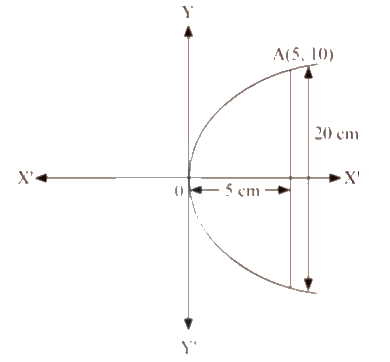The equation of the parabola is of the form $${y^2} = 4ax$$ (as it is opening to the right).

Since the parabola passes through point $$A\left( {5,10} \right)$$

Then,

\begin{align}&\Rightarrow {10^2} = 4a\left( 5 \right)\\&\Rightarrow 100 = 20a\\&\Rightarrow a = \frac{{100}}{{20}} = 5\end{align}

Therefore, the focus of the parabola is $$\left( {a,0} \right) \Rightarrow \left( {5,0} \right)$$, which is the mid-point of the diameter.

Hence, the focus of the reflector is at the mid-point of the diameter.

## Chapter 11 Ex.11.ME Question 2

An arch is in the form of a parabola with its axis vertical. The arch is $$10$$ m high and $$5$$ m wide at the base. How wide is it $$2$$ m from the vertex of the parabola?

### Solution

The origin of the coordinate plane is taken at the vertex of the parabolic reflector in such a way that the axis of the reflector is along the positive y-axis.

This can be diagrammatically represented as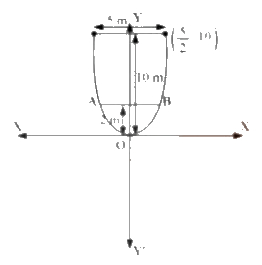The equation of the parabola is of the form $${x^2} = 4ay$$ (as it is opening upwards).

It can be clearly seen that the parabola passes through point $$\left( {\frac{5}{2},10} \right)$$

Hence,

\begin{align}&\Rightarrow {\left( {\frac{5}{2}} \right)^2} = 4a\left( {10} \right)\\&\Rightarrow a = \frac{{25}}{{4 \times 4 \times 10}} = \frac{5}{{32}}\end{align}

Therefore, the arch is in the form of a parabola whose equation is $${x^2} = \frac{5}{8}y$$

When $$y = 2$$

\begin{align}&\Rightarrow {x^2} = \frac{5}{8} \times 2\\&\Rightarrow {x^2} = \frac{5}{4}\\&\Rightarrow x = \sqrt {\frac{5}{4}} \end{align}

Therefore,

\begin{align}AB &= 2 \times 1.118\;{\rm{m}}\;\left( {{\rm{approx}}.} \right)\\&= 2.236\;{\rm{m}}\left( {{\rm{approx}}.} \right)\end{align}

Hence, when the arch is $$2$$m from the vertex of the parabola, its width is approximately $$2.236$$ m.

## Chapter 11 Ex.11.ME Question 3

The cable of a uniformly loaded suspension bridge hangs in the form of a parabola. The roadway which is horizontal and $$100$$ m long is supported by vertical wires attached to the cable, the longest wire being $$30$$ m and the shortest being $$6$$ m. Find the length of a supporting wire attached to the roadway $$18$$ m from the middle.

### Solution

The vertex is at the lowest point of the cable. The origin of the coordinate plane is taken as the vertex of the parabola, while its vertical axis is taken along the positive$$y -$$axis. This can be diagrammatically represented as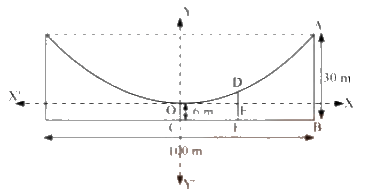Here, AB and OC are the longest and the shortest wires, respectively, attached to the cable. DF is the supporting wire attached to the roadway, 18 m from the middle.

Here, $$AB = 30\;m,$$$$OC = 6m$$ and $$BC = \frac{{100m}}{2} = 50m$$

The equation of the parabola is of the form $${x^2} = 4ay$$ (as it is opening upwards).

The coordinates of point A are$$\left( {50,30 - 6} \right) \Rightarrow \left( {50,24} \right)$$.

Since $$A\left( {50,24} \right)$$ is a point on the parabola,

Then,

\begin{align}&\Rightarrow {\left( {50} \right)^2} = 4a\left( {24} \right)\\&\Rightarrow a = \frac{{50 \times 50}}{{4 \times 24}}\\&\Rightarrow a = \frac{{625}}{{24}}\end{align}

Therefore, equation of the parabola,

\begin{align}&\Rightarrow {x^2} = 4 \times \frac{{625}}{{24}} \times y\\&\Rightarrow 6{x^2} = 625y\end{align}

The x-coordinate of point D is $$18$$.

Hence, at $$x = 18$$

\begin{align}&\Rightarrow 6{\left( {18} \right)^2} = 625y\\&\Rightarrow y = \frac{{6 \times 18 \times 18}}{{625}}\\&\Rightarrow y = 3.11\left( {{\rm{approx}}.} \right)\end{align}

Therefore,

\begin{align}DE&= 3.11m\\DF&= DE + EF\\&= 3.11m + 6m\\&= 9.11m\end{align}

Thus, the length of the supporting wire attached to the roadway $$18$$ m from the middle is approximately $$9.11$$ m.

## Chapter 11 Ex.11.ME Question 4

An arch is in the form of a semi-ellipse. It is $$8$$ m wide and $$2$$ m high at the centre. Find the height of the arch at a point $$1.5$$ m from one end.

### Solution

Since the height and width of the arc from the centre is $$2$$ m and $$8$$ m respectively, it is clear that the length of the major axis is 8 m, while the length of the semi-minor axis is $$2$$ m. The origin of the coordinate plane is taken as the centre of the ellipse, while the major axis is taken along the x-axis. Hence, the semi-ellipse can be diagrammatically represented as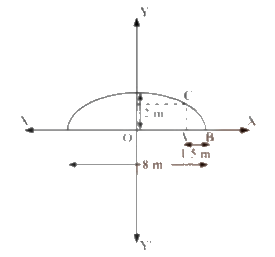The equation of the semi-ellipse will be of the form $$\frac{{{x^2}}}{{{a^2}}} + \frac{{{y^2}}}{{{b^2}}} = 1,\;y \ge 0$$, where $$a$$ is the semi-major axis

Accordingly,

$$\Rightarrow 2a = 8 \Rightarrow a = 4$$ and $$\Rightarrow b = 2$$

Therefore, the equation of the semi-ellipse is

$\frac{{{x^2}}}{{16}} + \frac{{{y^2}}}{4} = 1,\;y \ge 0\;\;\;\;\;\;\;\;\;\;\;\;\;\;\;\;\;\;\; \ldots \left( 1 \right)$

Let A be a point on the major axis such that $$AB = 1.5m$$.

Now, Draw $$AC \bot OB$$ .

$OA = \left( {4 - 1.5} \right)m = 2.5m$

The x-coordinate of point C is $$2.5$$

On substituting the value of $$x = 2.5$$ in equation $$\left( 1 \right)$$, we obtain

\begin{align}&\Rightarrow \frac{{{{\left( {2.5} \right)}^2}}}{{16}} + \frac{{{y^2}}}{4} = 1\\&\Rightarrow \frac{{6.25}}{{16}} + \frac{{{y^2}}}{4} = 1\\&\Rightarrow {y^2} = 4\left( {1 - \frac{{6.25}}{{16}}} \right)\\&\Rightarrow {y^2} = 4\left( {\frac{{9.75}}{{16}}} \right)\\&\Rightarrow {y^2} = 2.4375\\&\Rightarrow y = 1.56\;\left( \text{approx.} \right)\end{align}

Therefore, $$AC = 1.56m$$

Thus, the height of the arch at a point $$1.5$$ m from one end is approximately $$1.56$$ m.

## Chapter 11 Ex.11.ME Question 5

A rod of length $$12$$ cm moves with its ends always touching the coordinate axes. Determine the equation of the locus of a point P on the rod, which is $$3$$ cm from the end in contact with the x-axis.

### Solution

Let $$AB = 12cm$$ be the rod making an angle $${\rm{\theta }}$$ with $$OX$$ and $$P\left( {x,y} \right)$$ be the point on it such that $$AP = 3cm$$

Then,

\begin{align}PB&= AB - AP\\&= \left( {12 - 3} \right)cm\\&= 9cm\end{align}

From P, draw $$PQ \bot OY$$ and $$PR \bot OX$$.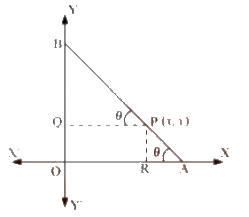In $$\Delta PBQ;\;\cos \theta = \frac{{PQ}}{{PB}} = \frac{x}{9}$$

In $$\Delta PRA;\;\sin \theta = \frac{{PR}}{{PA}} = \frac{y}{3}$$

Since, $${\sin ^2}\theta + {\cos ^2}\theta = 1$$,

\begin{align}{\left( {\frac{y}{3}} \right)^2} + {\left( {\frac{x}{9}} \right)^2} &= 1\\\Rightarrow {\frac{x}{{81}}^2} + \frac{{{y^2}}}{9} &= 1\end{align}

Thus, the equation of the locus of point P on the rod is $${\frac{x}{{81}}^2} + \frac{{{y^2}}}{9} = 1$$

## Chapter 11 Ex.11.ME Question 6

Find the area of the triangle formed by the lines joining the vertex of the parabola $${x^2} = \;12y$$ to the ends of its latus rectum.

### Solution

The given parabola is $${x^2} = \;12y$$.

On comparing this equation with $${x^2} = \;4ay$$, we obtain $$4a = 12 \Rightarrow a = 3$$

Therefore,

The coordinates of foci are $$\Rightarrow S\left( {0,3} \right)$$

Let AB be the latus rectum of the given parabola.

The given parabola can be roughly drawn as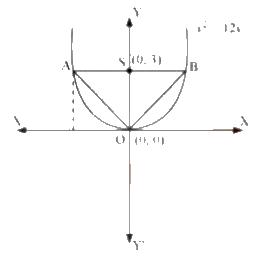At $$y = 3$$

\begin{align}&\Rightarrow {x^2} = 12\left( 3 \right)\\&\Rightarrow {x^2} = 36\\&\Rightarrow x = \pm 6\end{align}

Hence,

The coordinates of A are $$\left( { - 6,3} \right)$$, while the coordinates of B are $$\left( {6,3} \right)$$

Therefore, the vertices of $$\Delta AOB$$ are $$O\left( {0,0} \right),A\left( { - 6,3} \right)$$ and $$B\left( {6,3} \right)$$

\begin{align}ar\left( {\Delta AOB} \right) &= \frac{1}{2}\left| {0\left( {3 - 3} \right) + \left( { - 6} \right)\left( {3 - 0} \right) + 6\left( {0 - 3} \right)} \right|uni{t^2}\\&= \frac{1}{2}\left| {\left( { - 6} \right)\left( 3 \right) + 6\left( { - 3} \right)} \right|uni{t^2}\\&= \frac{1}{2}\left| { - 18 - 18} \right|uni{t^2}\\&= \frac{1}{2}\left| { - 36} \right|uni{t^2}\\&= \frac{1}{2} \times 36uni{t^2}\\&= 18uni{t^2}\end{align}

Thus, the required area of the triangle is $$18{\rm{ uni}}{{\rm{t}}^2}$$.

## Chapter 11 Ex.11.ME Question 7

A man running a racecourse notes that the sum of the distances from the two flag posts from him is always $$10$$ m and the distance between the flag posts is $$8$$m. Find the equation of the posts traced by man.

### Solution

Let A and B be the positions of the two flag posts and $$P\left( {x,y} \right)$$ be the position of the man.

Accordingly,

$$PA + PB = 10$$.

We know that if a point moves in a plane in such a way that the sum of its distances from two fixed points is constant, then the path is an ellipse and this constant value is equal to the length of the major axis of the ellipse.

Therefore, the path described by the man is an ellipse where the length of the major axis is $$10$$ m, while points $$A$$ and $$B$$ are the foci.

Taking the origin of the coordinate plane as the centre of the ellipse, while taking the major axis along the $$x-$$axis, the ellipse can be diagrammatically represented as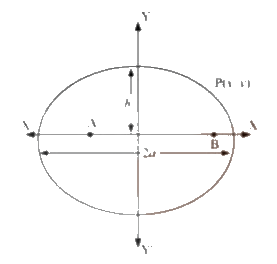The equation of the ellipse will be of the form $$\frac{{{x^2}}}{{{a^2}}} + \frac{{{y^2}}}{{{b^2}}} = 1$$, where $$a$$ is the semi-major axis

Accordingly,

\begin{align}&\Rightarrow 2a = 10\\&\Rightarrow a = 5\end{align}

Distance between the foci

\begin{align}&\Rightarrow 2c = 8\\&\Rightarrow c = 4\end{align}

On using the relation $$c = \sqrt {{a^2} - {b^2}}$$, we obtain

\begin{align}&\Rightarrow 4 = \sqrt {25 - {b^2}} \\&\Rightarrow 16 = 25 - {b^2}\\&\Rightarrow {b^2} = 25 - 16\\&\Rightarrow {b^2} = 9\\&\Rightarrow b = 3\end{align}

Thus, the equation of the path traced by the man is $$\frac{{{x^2}}}{{25}} + \frac{{{y^2}}}{9} = 1$$

## Chapter 11 Ex.11.ME Question 8

An equilateral triangle is inscribed in the parabola $${y^2} = \;4ax$$, where one vertex is at the vertex of the parabola. Find the length of the side of the triangle.

### Solution

Let $$\Delta OAB$$ be the equilateral triangle inscribed in the parabola $${y^2} = \;4ax$$.

Let AB intersect the $$x-$$axis at point $$C$$.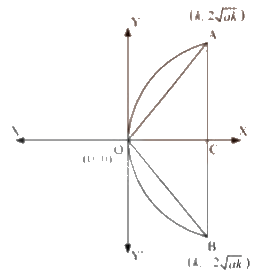Let $$OC = k$$

From the equation of the given parabola, we have

\begin{align}&\Rightarrow {y^2} = 4ak\\&\Rightarrow y = \pm 2\sqrt {ak} \end{align}

Therefore, the respective coordinates of points A and B are $$\left( {k,2\sqrt {ak} } \right)$$ and $$\left( {k, - 2\sqrt {ak} } \right)$$

Hence,

\begin{align}AB &= AC + CB\\&= 2\sqrt {ak} + 2\sqrt {ak} \\&= 4\sqrt {ak} \end{align}

Since, $$\Delta OAB$$ is an equilateral triangle,

\begin{align}&\Rightarrow O{A^2} = A{B^2}\\&\Rightarrow O{C^2} + A{C^2} = A{B^2}\end{align}

Therefore,

\begin{align}&\Rightarrow {k^2} + {\left( {2\sqrt {ak} } \right)^2} = {\left( {4\sqrt {ak} } \right)^2}\\&\Rightarrow {k^2} + 4ak = 16ak\\&\Rightarrow {k^2} = 12ak\\&\Rightarrow k = 12a\end{align}

Hence,

\begin{align}AB& = 4\sqrt {ak} = 4\sqrt {a \times 12a} \\&= 4\sqrt {12{a^2}} \\&= 8\sqrt 3 a\end{align}

Thus, the side of the equilateral triangle inscribed in the parabola $${y^2} = \;4ax$$ is $$8\sqrt 3 a$$.

Instant doubt clearing with Cuemath Advanced Math Program